Question

# 2. A unity feedback system has the following open-loop transfer function -0.5s + 0.5 G(s)i a)...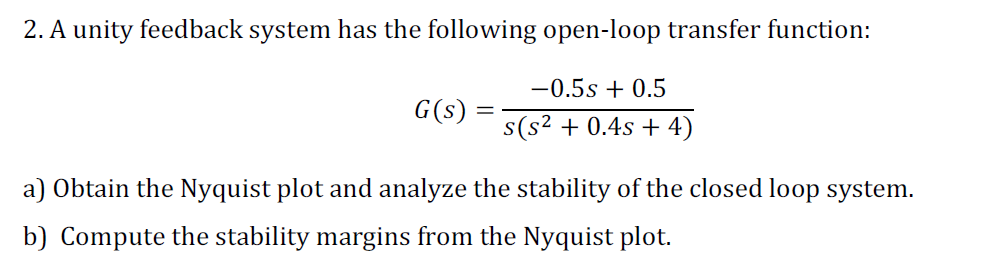2. A unity feedback system has the following open-loop transfer function -0.5s + 0.5 G(s)i a) Obtain the Nyquist plot and analyze the stability of the closed loop system b) Compute the stability margins from the Nyquist plot.

a) for unity feedback system H(s)=1

G(s)H(s)=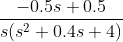Plot nyquist plot in Matlab using commands

>> H = tf([-0.5 0.5],[1 0.4 4 1]);
>> nyquist(H)

Nyquist plot :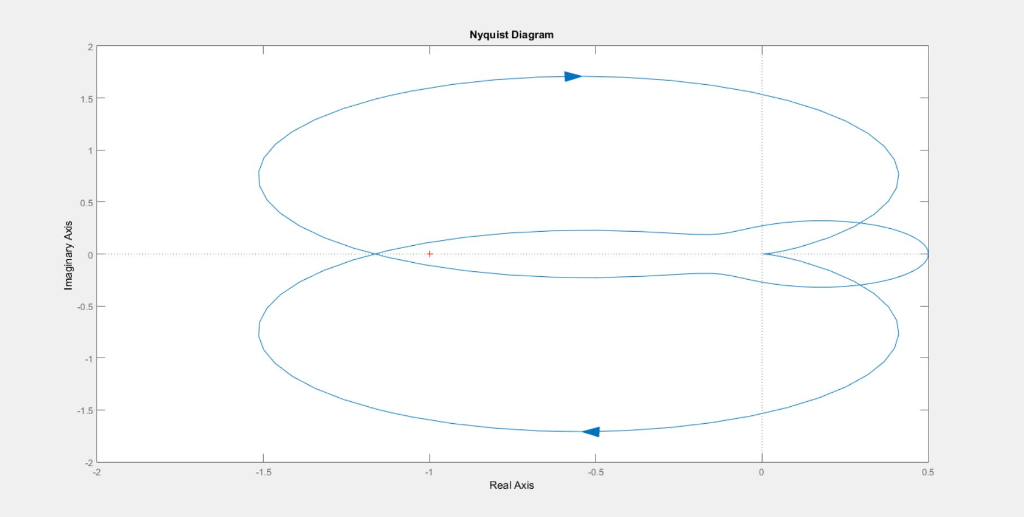b)

For stability margins

type command in matlab

>> margin(H)

and you will get the stability margins plot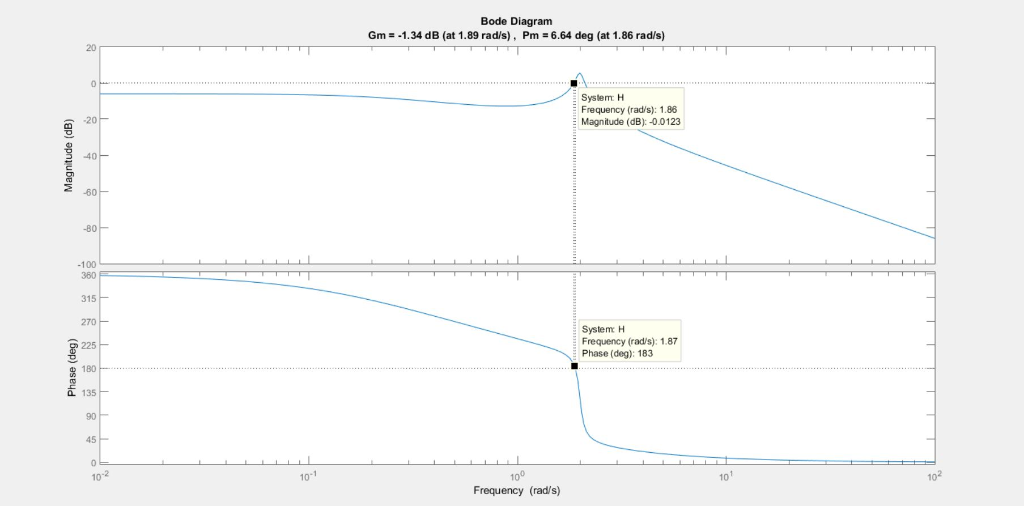#### Earn Coins

Coins can be redeemed for fabulous gifts.

Similar Homework Help Questions
• ### 2. Given a unity feedback system with open-loop transfer function s+40s-I) a) For K-1, derive the...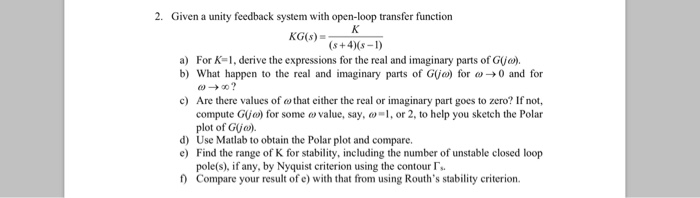2. Given a unity feedback system with open-loop transfer function s+40s-I) a) For K-1, derive the expressions for the real and imaginary parts of G(jo). b) What happen to the real and imaginary parts of G(jo) for ω 0 and for Are there values of ω that either the real or imaginary part goes to zero? If not, compute Gijo) for some ovalue, say,, or 2, to help you sketch the Polar plot of Gja). c) d) Use Matlab to...

• ### 4. Consider a unity-feedback control system with the following open-loop transfer function: G(s)3 Sketch a Nyquist...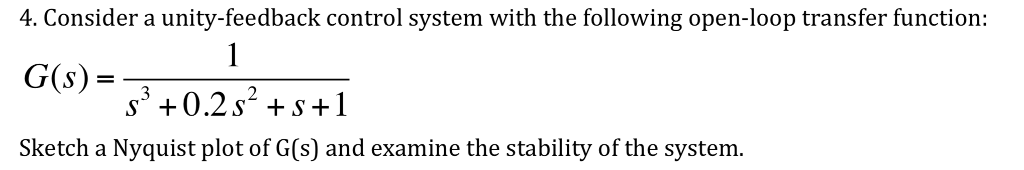4. Consider a unity-feedback control system with the following open-loop transfer function: G(s)3 Sketch a Nyquist plot of G(s) and examine the stability of the system.

• ### 1. Given a unity feedback system with the open-loop transfer function s(0.5s +1) .design a lead c...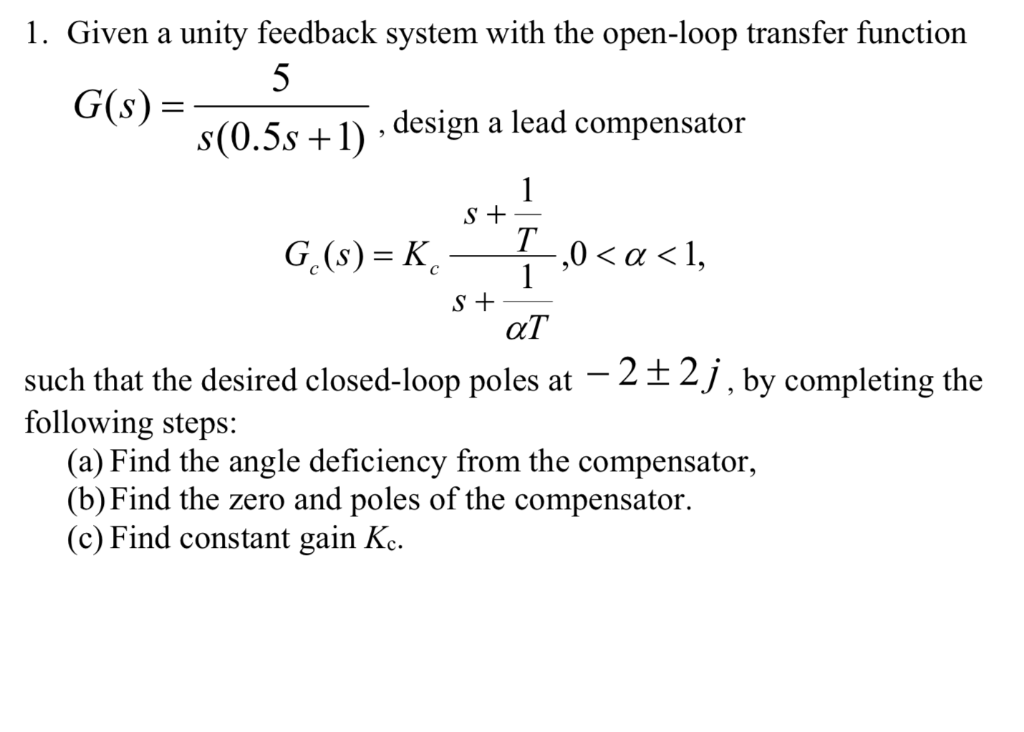1. Given a unity feedback system with the open-loop transfer function s(0.5s +1) .design a lead compensator ,0 〈 α 〈 1, such that the desired closed-loop poles at -2+2j following steps: J, by completing the (a) Find the angle deficiency from the compensator, (b)Find the zero and poles of the compensator (c) Find constant gain Kc. 1. Given a unity feedback system with the open-loop transfer function s(0.5s +1) .design a lead compensator ,0 〈 α 〈 1, such...

• ### A unity feedback control system has the following open loop transfer function, K L(s) .If K 10, evaluate the stability...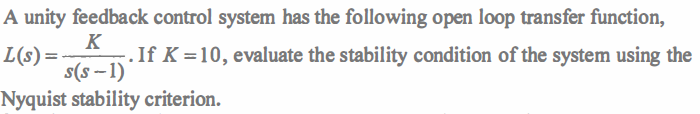A unity feedback control system has the following open loop transfer function, K L(s) .If K 10, evaluate the stability condition of the system using the s(s-1) Nyquist stability criterion. A unity feedback control system has the following open loop transfer function, K L(s) .If K 10, evaluate the stability condition of the system using the s(s-1) Nyquist stability criterion.

• ### Consider a unity-feedback control system with the following open-loop transfer G(s)

Consider a unity-feedback control system with the following open-loop transfer G(s) = 1/(s3+0.2s2 +s+1) Draw a Nyquist plot of G(s). You may draw the simple Nyquist plot

• ### 1. A unity feedback system has open-loop transfer function given by an 100 G(s)s2)(s +4) a....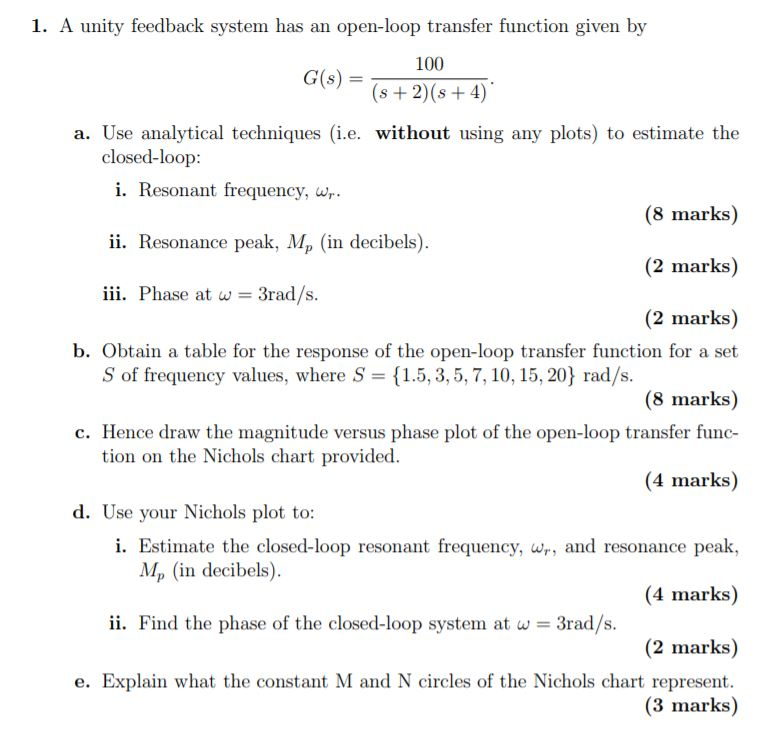1. A unity feedback system has open-loop transfer function given by an 100 G(s)s2)(s +4) a. Use analytical techniques (i.e. without using any plots) to estimate the closed-loop: i. Resonant frequency, w (8 marks) ii. Resonance peak, Mp (in decibels) (2 marks) i. Phase at w = 3rad/s (2 marks) b. Obtain a table for the response of the open-loop transfer function for a set S of frequency values, where S {1.5,3,5,7, 10, 15, 20} rad/s (8 marks) Hence draw...

• ### 1. A unity feedback system has open-loop transfer function given by an 100 G(s)s2)(s +4) a....1. A unity feedback system has open-loop transfer function given by an 100 G(s)s2)(s +4) a. Use analytical techniques (i.e. without using any plots) to estimate the closed-loop: i. Resonant frequency, w (8 marks) ii. Resonance peak, Mp (in decibels) (2 marks) i. Phase at w = 3rad/s (2 marks) b. Obtain a table for the response of the open-loop transfer function for a set S of frequency values, where S {1.5,3,5,7, 10, 15, 20} rad/s (8 marks) Hence draw...

• ### A unity gain negative feedback system has an open-loop transfer function given by 4. s) =...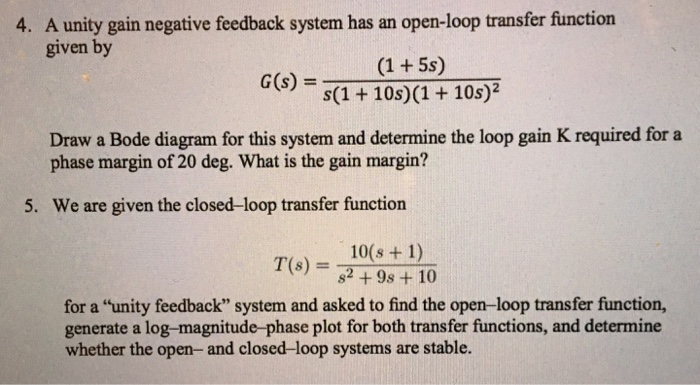A unity gain negative feedback system has an open-loop transfer function given by 4. s) = s(1 + 10s)(1 + 10s)? Draw a Bode diagram for this system and determine the loop gain K required for a phase margin of 20 deg. What is the gain margin? 5. We are given the closed-loop transfer function 10(s + 1) T(s) = 82+98+10 for a "unity feedback" system and asked to find the open-loop transfer function, generate a log-magnitude-phase plot for both...

• ### A unity feedback system has the following open-loop gain function 10 s(s+2) Use MATLAB to plot...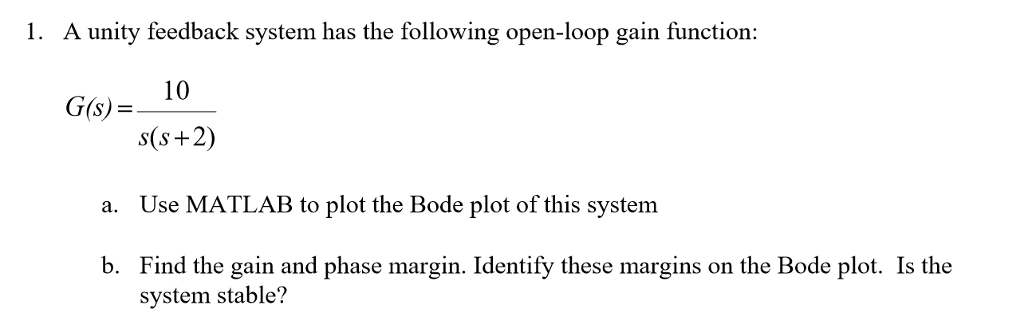A unity feedback system has the following open-loop gain function 10 s(s+2) Use MATLAB to plot the Bode plot of this system Find the gain and phase margin. Identify these margins on the Bode plot. Is the G(s) a. b. system stable?

• ### QUESTION 4 A unity feedback system has open-loop transfer function G(S). Polar plot of G(jw) is...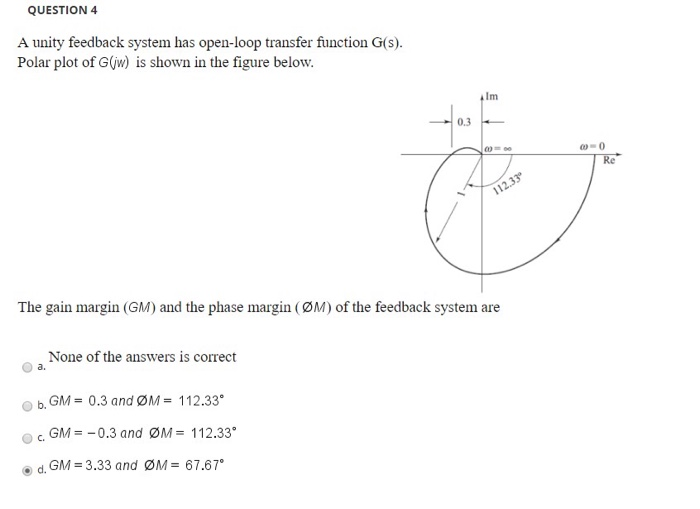QUESTION 4 A unity feedback system has open-loop transfer function G(S). Polar plot of G(jw) is shown in the figure below. The gain margin (GM) and the phase margin (ØM) of the feedback system are None of the answers is correct b. GM = 0.3 and ØM = 112.33º O GM = -0.3 and ØM = 112.33 d. GM = 3.33 and ØM = 67.67° QUESTION 8 A unity feedback system as open-loop transfer function G(s) = 5(1 +ST) The...